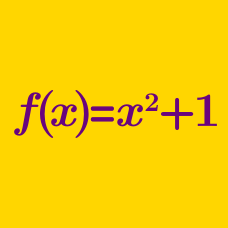Algebra

Function Problem Solving

If $f(x) = 3x - 3$ and $g(x) = -3x + 1$, what is the value of $a$ satisfying $\left(g \circ f^{-1}\right)(a) = 4?$

Details and assumptions

• $f^{-1}(x)$ denotes the inverse function of $f(x).$

For three functions $f$, $g$ and $h$ $f(x)=x-3, (h \circ g)(x)=6x+4.$ What is the value of $x$ that satisfies $(h \circ (g \circ f))(x)=52?$

Details and assumptions

Composite function $(h \circ g)(x)$ denotes $h(g(x))$.

For two sets $X=\{-2, -1, 0, 1, 2\}, Y=\{y \mid y \text{ is an integer}\},$ function $f: X \to Y$ is defined as $f(x)=\begin{cases} x+11 & \text{ if } x > 0 \\ -x^2+4 & \text{ if } x \leq 0. \end{cases}$ What is the sum of all the elements of the range of $f$?

If $\ X = \{ -5, 0, 5 \}$ and $Y = \{ y \mid -11 \leq y \leq 8,\ y \in \mathbf{Z} \}$, how many functions $f: X \rightarrow Y$ are there such that $x \cdot f(x)$ is a constant function for all elements of $x$ in $X$?

Details and assumptions

$\mathbf{Z}$ is the set of all integers.

Consider two functions $f(x)=ax+1, g(x)=-x-2.$ If it always holds that $f\circ g=g\circ f$, what is the value of the constant $a$?

×